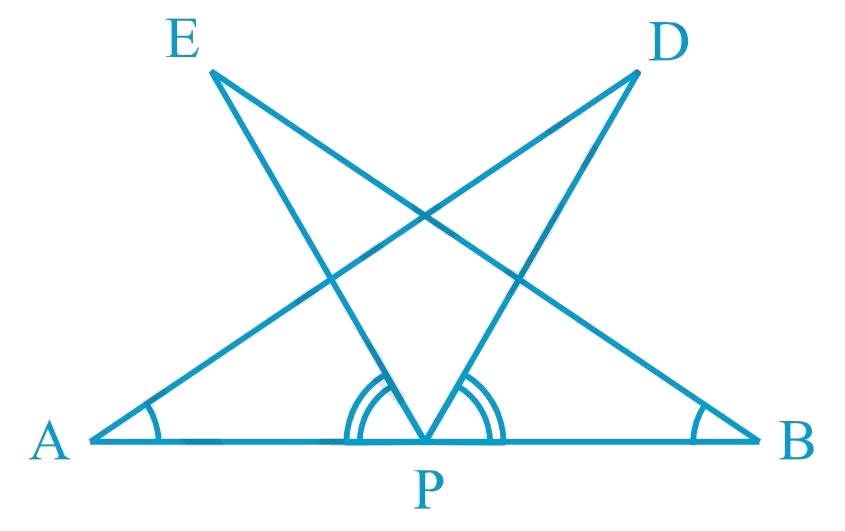# Ex.7.1 Q7 Triangles Solution - NCERT Maths Class 9

## Question

$$AB$$ is a line segment and $$P$$ is its mid-point. $$D$$ and $$E$$ are points on the same side of $$AB$$

such that $$\angle BAD = \angle ABE$$ and

$$\angle EPA = \angle DPB$$ (See the given figure).

Show that:

(i) $$\Delta DAP \cong \Delta EBP$$

(ii) $$AD = BE$$

Video Solution
Triangles
Ex 7.1 | Question 7

## Text Solution

What is Known?

$$P$$ is its mid-point of $$AB$$

$$\angle BAD = \angle ABE{\text{ }}and\;\angle EPA = \angle DPB$$,

To prove:

(i) $$\Delta DAP \cong \Delta EBP$$ and (ii) $$AD = BE$$ .

Reasoning:

We can show two triangles DAP and EBP congruent by using ASA congruency rule and then we can say corresponding parts of congruent triangles will be equal. To show both triangles congruent one pair of equal sides and one pair of equal angles are given and add angle EPD on both sides in given pair of angles EPA and angle DPB to find the other pair of angles APD and BPESteps:

It is given that

$$\angle EPA = \angle DPB$$

$$\angle EPA + \angle DPE = \angle DPB + \angle DPE$$

$$\therefore \angle DPA = \angle EPB$$

In $$\Delta DAP$$ and $$\Delta EBP$$,

\begin{align}\angle DAP&=\angle EBP\,\,\,(\text{Given})\,\\AP&=BP\,\\(P\,\text{is}\,\text{mid}\,&\text{point}\,\text{of}\,\text{AB})\,\\\\\angle DPA&=\angle EPB\,\,\,(\text{From}\,\text{above})\,\,\\\therefore \Delta DAP&\cong \text{ }\!\!~\!\!\text{ }\Delta EBP\,\\&(\text{ASAcongruencerule})\,\\\\\therefore AD&=BE\,(By\,CPCT)\end{align}

Video Solution
Triangles
Ex 7.1 | Question 7
Learn from the best math teachers and top your exams

• Live one on one classroom and doubt clearing
• Practice worksheets in and after class for conceptual clarity
• Personalized curriculum to keep up with school